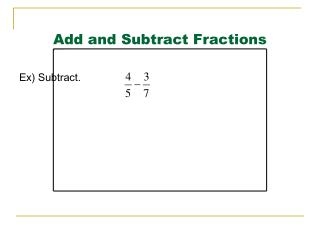DownloadDownload PresentationDownload Presentation- - - - - - - - - - - - - - - - - - - - - - - - - - - E N D - - - - - - - - - - - - - - - - - - - - - - - - - - -
##### Presentation Transcript

1. Add and Subtract Fractions Ex) Subtract.

2. Add and Subtract Fractions Ex) Subtract.

3. Add and Subtract Fractions Ex) Subtract.

4. Add and Subtract Fractions Ex) Subtract.

5. Adding and Subtracting Mixed Numbers

6. Adding and Subtracting Mixed Numbers • Ex 2) Subtract.

7. Adding and Subtracting Mixed Numbers • Ex 2) Subtract.

8. Adding and Subtracting Mixed Numbers • Ex 2) Subtract.

9. Adding and Subtracting Mixed Numbers • Ex 2) Subtract.

10. Adding and Subtracting Mixed Numbers • Ex 2) Subtract.

11. Adding and Subtracting Mixed Numbers • Ex 2) Subtract.

12. Adding and Subtracting Mixed Numbers • Ex 2) Subtract.

13. Adding and Subtracting Mixed Numbers • Ex 2) Subtract.